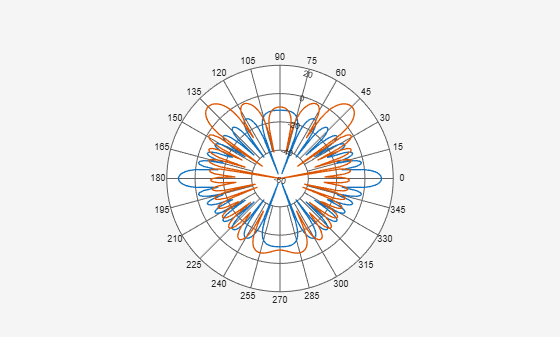Main Content

# findLobes

Class: polarpattern

Main, back, and side lobe data

## Syntax

``L = findLobes(p)``
``L = findLobes(p,index)``

## Description

example

````L = findLobes(p)` returns a structure, `L`, defining the main, back, and side lobes of the antenna or array radiation pattern in the specified polar plot, `p`.```

example

````L = findLobes(p,index)` returns the radiation pattern lobes from the data set specified in `index`.```

## Input Arguments

expand all

Polar plot, specified as a scalar handle.

Index of data set, specified as a scalar.

## Examples

expand all

Create a 15-element ULA of isotropic antenna with elements spaced one-half wavelength apart. Plot the directivity of the array at 20 GHz. Then, find the mainlobe, sidelobe, and backlobe directions of the array pattern.

```fc = 20.0e9; c = physconst('Lightspeed'); lam = c/fc; angs = [-180:1:180]; antenna = phased.IsotropicAntennaElement('FrequencyRange',[1.0e9,100.0e9]); array = phased.ULA('Element',antenna,'NumElements',15,'ElementSpacing',lam/2); a = pattern(array,fc,angs,0); P = polarpattern(angs,a);````L = findLobes(P)`
```L = struct with fields: mainLobe: [1x1 struct] backLobe: [1x1 struct] sideLobes: [1x1 struct] FB: 0 SLL: 0 HPBW: 8.0000 FNBW: 16.0000 FBIdx: [181 1] SLLIdx: [181 361] HPBWIdx: [357 5] HPBWAng: [176 -176] FNBWIdx: [173 189] ```

Create a 15-element ULA of isotropic antenna with elements spaced one-half wavelength apart. Plot the directivity of the array at 20 GHz. Then steer the array to 45° azimuth and plot the directivity. Then, find the mainlobe, sidelobe, and backlobe directions of the array pattern.

```fc = 20.0e9; c = physconst('Lightspeed'); lam = c/fc; angs = [-180:1:180]; antenna = phased.IsotropicAntennaElement('FrequencyRange',[1.0e9,100.0e9]); array = phased.ULA('Element',antenna,'NumElements',15,'ElementSpacing',lam/2); a = pattern(array,fc,angs,0); P = polarpattern(angs,a);```Steer the array to 45&deg azimuth and add the steered pattern to the polar plot.

```steervec = phased.SteeringVector('SensorArray',array,'PropagationSpeed',c); sv = steervec(fc,[45;0]); a1 = pattern(array,fc,angs,0,'Weights',sv); add(P,angs,a1);```Find the lobes of the steered pattern.

```L = findLobes(P,2); L.mainLobe```
```ans = struct with fields: index: 226 magnitude: 11.7609 angle: 45 extent: [216 238] ```

## See Also

Introduced in R2016a

## Support

#### Exploring Hybrid Beamforming Architectures for 5G Systems

Download white paper